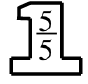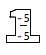### Home > MC1 > Chapter 8 > Lesson 8.2.4 > Problem8-67

8-67.

For each of the following pairs of fractions, complete the fraction on the right so that the two fractions are equivalent. Using a Giant One might be helpful.

1.  $\frac { 30 } { 35 } = \frac { 6 } { \square }$

The Giant One for this problem could look like this:$\frac{30}{35}=\frac{6}{7}$

since $35$ divided by $5$ is $7$.

1.  $\frac { 8 } { 40 } = \frac { \square } { 5 }$

Make a Giant One like in part (a), if that is helpful for you.

1.  $\frac { - 15 } { - 20 } = \frac { \square } { 4 }$The Giant One for this problem could look like this: